Postscript version of these notes

STAT 804

Lecture 16 Notes

Distribution theory for sample autocovariances

The simplest statistic to consider is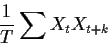where the sum extends over those t for which the data are available. If the series has mean 0 then the expected value of this statistic is simply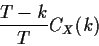which differs negligibly for T large compared to k from CX(k). To compute the variance we begin with the second moment which is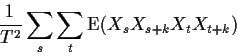The expectations in question involve the fourth order product moments of X and depend on the distribution of the X's and not just on CX. However, for the interesting case of white noise, we can compute the expected value. For k> 0 you may assume that s<t or s=t since the s> t cases can be figured out by swapping s and t in the s<t case. For s<t the variable Xs is independent of all 3 of Xs+k, Xt and Xt+k. Thus the expectation factors into something containing the factor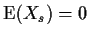. For s=t, we get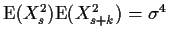. and so the second moment is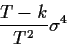This is also the variance since, for k> 0 and for white noise, CX(k)=0.

For k=0 and s <t or s> t the expectation is simply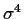while for s=t we get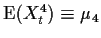. Thus the variance of the sample variance (when the mean is known to be 0) is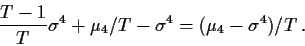For the normal distribution the fourth moment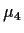is given simply by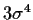.

Having computed the variance it is usual to look at the large sample distribution theory. For k=0 the usual central limit theorem applies to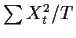(in the case of white noise) to prove that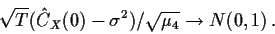The presence ofin the formula shows that the approximation is quite sensitive to the assumption of normality.

For k> 0 the theorem needed is called the m-dependent central limit theorem; it shows that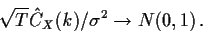In each of these cases the assertion is simply that the statistic in question divided by its standard deviation has an approximate normal distribution.

The sample autocorrelation at lag k is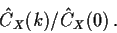For k> 0 we can apply Slutsky's theorem to conclude that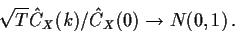This justifies drawing lines at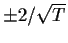to carry out a 95% test of the hypothesis that the X series is white noise based on the kth sample autocorrelation.

It is possible to verify that subtraction of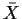from the observations before computing the sample covariances does not change the large sample approximations, although it does affect the exact formulas for moments.

When the X series is actually not white noise the situation is more complicated. Consider as an example the model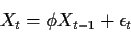with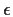being white noise. Taking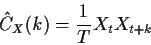we find that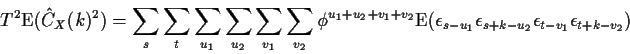The expectation is 0 unless either all 4 indices on the's are the same or the indices come in two pairs of equal values. The first case requires u1=u2-k and v1=v2-k and then s-u1=t-v1. The second case requires one of three pairs of equalities: s-u1=t-v1 and s-u2 = t-v2 or s-u1=t+k-v2 and s+k-u2 = t-v1 or s-u1=s+k-u2 and t-v1 = t-+k-v2 along with the restriction that the four indices not all be equal. The actual moment is thenwhen all four indices are equal andwhen there are two pairs. It is now possible to do the sum using geometric series identities and compute the variance of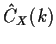. It is not particularly enlightening to finish the calculation in detail.

There are versions of the central limit theorem called mixing central limit theorems which can be used for ARMA(p,q) processes in order to conclude that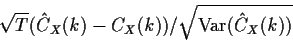has asymptotically a standard normal distribution and that the same is true when the standard deviation in the denominator is replaced by an estimate. To get from this to distribution theory for the sample autocorrelation is easiest when the true autocorrelation is 0.

The general tactic is the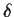method or Taylor expansion. In this case for each sample size T you have two estimates, say NT and DTof two parameters. You want distribution theory for the ratio RT = NT/DT. The idea is to write RT=f(NT,DT) where f(x,y)=x/y and then make use of the fact that NT and DT are close to the parameters they are estimates of. In our case NTis the sample autocovariance at lag k which is close to the true autocovariance CX(k) while the denominator DT is the sample autocovariance at lag 0, a consistent estimator of CX(0).

Write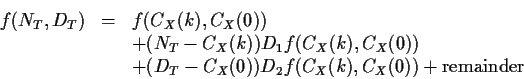If we can use a central limit theorem to conclude that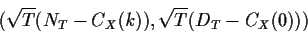has an approximately bivariate normal distribution and if we can neglect the remainder term then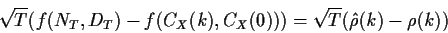has approximately a normal distribution. The notation here is that Dj denotes differentiation with respect to the jth argument of f. For f(x,y) = x/y we have D1f = 1/y and D2f = -x/y2. When CX(k)=0 the term involving D2f vanishes and we simply get the assertion that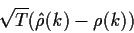has the same asymptotic normal distribution as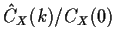.

Similar ideas can be used for the estimated sample partial ACF.

Portmanteau tests

In order to test the hypothesis that a series is white noise using the distribution theory just given, you have to produce a single statistic to base youre test on. Rather than pick a single value of k the suggestion has been made to consider a sum of squares or a weighted sum of squares of the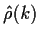.

A typical statistic is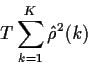which, for white noise, has approximately a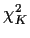distribution. (This fact relies on an extension of the previous computations to conclude that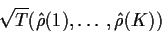has approximately a standard multivariate distribution. This, in turn, relies on computation of the covariance between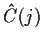and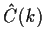.)

When the parameters in an ARMA(p,q) have been estimated by maximum likelihood the degrees of freedom must be adjusted to K-p-q. The resulting test is the Box-Pierce test; a refined version which takes better account of finite sample properties is the Box-Pierce-Ljung test. S-Plus plots the P-values from these tests for 1 through 10 degrees of freedom as part of the output of arima.diag.Richard Lockhart
1999-10-13